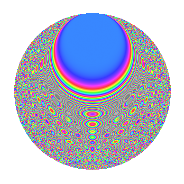# Properties

 Label 3234.2.nLevel 3234 Weight 2 Character orbit n Rep. character $$\chi_{3234}(263,\cdot)$$ Character field $$\Q(\zeta_{6})$$ Dimension 320 Sturm bound 1344

# Related objects

## Defining parameters

 Level: $$N$$ $$=$$ $$3234 = 2 \cdot 3 \cdot 7^{2} \cdot 11$$ Weight: $$k$$ $$=$$ $$2$$ Character orbit: $$[\chi]$$ $$=$$ 3234.n (of order $$6$$ and degree $$2$$) Character conductor: $$\operatorname{cond}(\chi)$$ $$=$$ $$231$$ Character field: $$\Q(\zeta_{6})$$ Sturm bound: $$1344$$

## Dimensions

The following table gives the dimensions of various subspaces of $$M_{2}(3234, [\chi])$$.

Total New Old
Modular forms 1408 320 1088
Cusp forms 1280 320 960
Eisenstein series 128 0 128

## Trace form

 $$320q - 160q^{4} - 16q^{9} + O(q^{10})$$ $$320q - 160q^{4} - 16q^{9} + 32q^{15} - 160q^{16} + 12q^{22} + 140q^{25} + 24q^{27} + 4q^{31} - 26q^{33} + 8q^{34} + 32q^{36} + 12q^{37} + 56q^{45} - 84q^{55} + 32q^{58} - 16q^{60} + 320q^{64} - 8q^{66} - 8q^{67} + 64q^{69} + 52q^{75} + 64q^{78} + 24q^{81} - 16q^{82} - 6q^{88} + 68q^{93} - 32q^{97} + 136q^{99} + O(q^{100})$$

## Decomposition of $$S_{2}^{\mathrm{new}}(3234, [\chi])$$ into newform subspaces

The newforms in this space have not yet been added to the LMFDB.

## Decomposition of $$S_{2}^{\mathrm{old}}(3234, [\chi])$$ into lower level spaces

$$S_{2}^{\mathrm{old}}(3234, [\chi]) \cong$$ $$S_{2}^{\mathrm{new}}(231, [\chi])$$$$^{\oplus 4}$$$$\oplus$$$$S_{2}^{\mathrm{new}}(462, [\chi])$$$$^{\oplus 2}$$$$\oplus$$$$S_{2}^{\mathrm{new}}(1617, [\chi])$$$$^{\oplus 2}$$

## Hecke characteristic polynomials

There are no characteristic polynomials of Hecke operators in the database